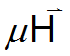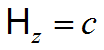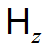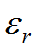﻿ Technical Notes > Applications in Electromagnetics > Waveguides > Material Interfaces

Material Interfaces

Navigation:  Technical Notes > Applications in Electromagnetics > Waveguides >

Material InterfacesAt a material interface, Maxwell’s equations require that the tangential components ofandand the normal components ofandmust be continuous.

The tangential continuity of componentsandis automatically satisfied, because FlexPDE stores only a single value of variables at the interface.

Continuity of, which is always tangential, requires, using (3.12),.

Continuity ofrequires.

At internal boundaries, and in the absence of Natural specification, FlexPDE will consider the quantities represented by the Natural BC to be continuous.

From (3.16) it is clear that at vertical interfaces, the continuity ofwill be satisfied by theequation, while for horizontal interfaces, it will be satisfied by theequation.

If all material discontinuities occur at vertical faces andis piecewise constant, we can multiply theequation by, and continuity ofwill be satisfied.  Similarly, if all material discontinuities occur at horizontal faces andis piecewise constant, we can multiply theequation by, and continuity ofwill be satisfied.

Clearly, at an internal interface whereis continuous, the internal natural boundary condition reduces to zero, which is the default condition.

In the example which follows, we consider a simple 2x1 metal box with dielectric material in the left half.  Note that FlexPDE will compute the eigenvalues with lowest magnitude, regardless of sign, while negative eigenvalues correspond to modes with propagation constants below cutoff, and are therefore not physically realizable.

Descriptor 3.2 Filledguide.pde

title "Filled Waveguide"

{ This problem is taken from Fernandez and Lu,

"Microwave and Optical Waveguide Analysis by the Finite Element Method" page 138. }

select

modes = 5     { This is the number of Eigenvalues desired. }

ngrid = 30

overshoot = 1e-9    { request very tight Conjugate Gradient convergence }

variables

Hx, Hy

definitions

cm = 0.01           ! conversion from cm to meters

b = 1*cm             ! box height

L = 2*b               ! box width

epsr                 ! values will be given by region

epsr1=1        epsr2=1.5

eps0 = 8.85e-12

mu0 = 4e-7*pi

c =  1/sqrt(mu0*eps0) ! light speed

k0b = 4                     ! normalized driving frequency

k0 = k0b/b

k02 = k0^2           ! k0^2=omega^2*mu0*eps0

! terms used in equations and BC’s

curlh = dx(Hy)-dy(Hx)

divh = dx(Hx)+dy(Hy)

! the solution generates some negative eigenvalues, so we shift the eigenvalues to a range where only the

! positive values appear

shift = 200000

true_lambda=lambda+shift

! extract the propagation wave number kz

kz = if(true_lambda>0)then sqrt(true_lambda) else -sqrt(abs(true_lambda))

equations

! Hx equation multiplied by epsr to enforce continuity of Hz

Hx: dx(divh) - dy(curlh) + k02*Hx*epsr - (lambda+shift)*Hx = 0

Hy: dx(curlh/epsr) + dy(divh)/epsr + k02*Hy - (lambda+shift)*Hy/epsr = 0

boundaries

region 1  epsr=epsr1

start(0,0)

natural(Hx) = 0 value(Hy)=0

line to (L,0)

value(Hx) = 0 value(Hy)=0 natural(Hy)=0

line to (L,b)

natural(Hx) = 0 value(Hy)=0

line to (0,b)

value(Hx) = 0 natural(Hy)=0

line to close

region 2  epsr=epsr2

start(b,b)

line to (0,b) to (0,0) to (b,0)

line to close

monitors

contour(Hx) range=(-1,1)

contour(Hy) range=(-1,1)

plots

contour(Hx) range=(-1,1) report(k0) report(kz)

surface(Hx) range=(-1,1) report(k0) report(kz)

contour(Hy) range=(-1,1) report(k0) report(kz)

surface(Hy) range=(-1,1) report(k0) report(kz)

surface(divh) range=(-1,1) as "Hz" report(k0) report(kz)

surface(curlh/epsr) range=(-1,1) as "Ez" report(k0) report(kz)

summary export

report lambda

report shift

report true_lambda

report(k0)

report( if(true_lambda<0) then "*" else " ") ! mark negative eigenvalues

report(kz)

report(kz/k0)

end